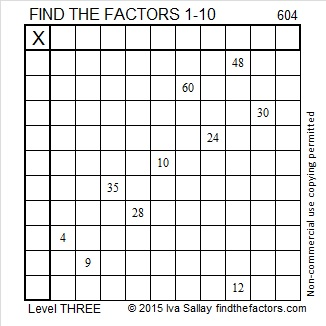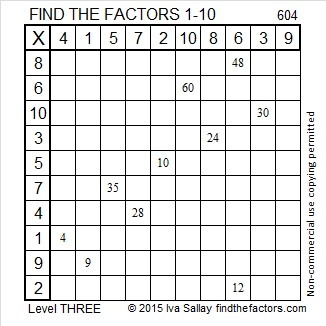# 604 and Level 3

604 is not a power of 2, can be evenly divided by 4, but not by 8, and 604 is greater than 28. Those three facts together mean that 604 can be written as the sum of 8 consecutive whole numbers. To find the 8 numbers first divide 604 by 8 to get 75 1/2, then add the 4 counting numbers before 75 1/2 to the 4 counting numbers after it:

72 + 73 + 74 + 75 + 76 + 77 + 78 + 79 = 604Print the puzzles or type the solution on this excel file: 10 Factors 2015-09-01

—————————————————————————————————

• 604 is a composite number.
• Prime factorization: 604 = 2 x 2 x 151, which can be written 604 = (2^2) x 151
• The exponents in the prime factorization are 2 and 1. Adding one to each and multiplying we get (2 + 1)(1 + 1) = 3 x 2  = 6. Therefore 604 has exactly 6 factors.
• Factors of 604: 1, 2, 4, 151, 302, 604
• Factor pairs: 604 = 1 x 604, 2 x 302, or 4 x 151
• Taking the factor pair with the largest square number factor, we get √604 = (√4)(√151) = 2√151 ≈ 24.576411—————————————————————————————————

A Logical Approach to solve a FIND THE FACTORS puzzle: Find the column or row with two clues and find their common factor. Write the corresponding factors in the factor column (1st column) and factor row (top row).  Because this is a level three puzzle, you have now written a factor at the top of the factor column. Continue to work from the top of the factor column to the bottom, finding factors and filling in the factor column and the factor row one cell at a time as you go.This site uses Akismet to reduce spam. Learn how your comment data is processed.